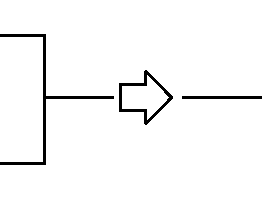# Parallel resistor calculator

## Chapter 5 - Resistor applications

Use this calculator to quickly calculate the equivalent resistance value of two resistors in parallel. Just enter the resistance value in ohms in the boxes for resistor 1 and 2. Automatically the equivalent resistance value will appear.The formula that is used to solve the problem is given by:If you want to have a more in-depth explanation on how to this formula works, then read the article resistors in parallel.

Notice that this calculator is not only valid for resistors. You can also calculate equivalent values for other math problems. Some examples are inductors in parallel, capacitors in series, or the spring stiffness of two springs in series.

• Share